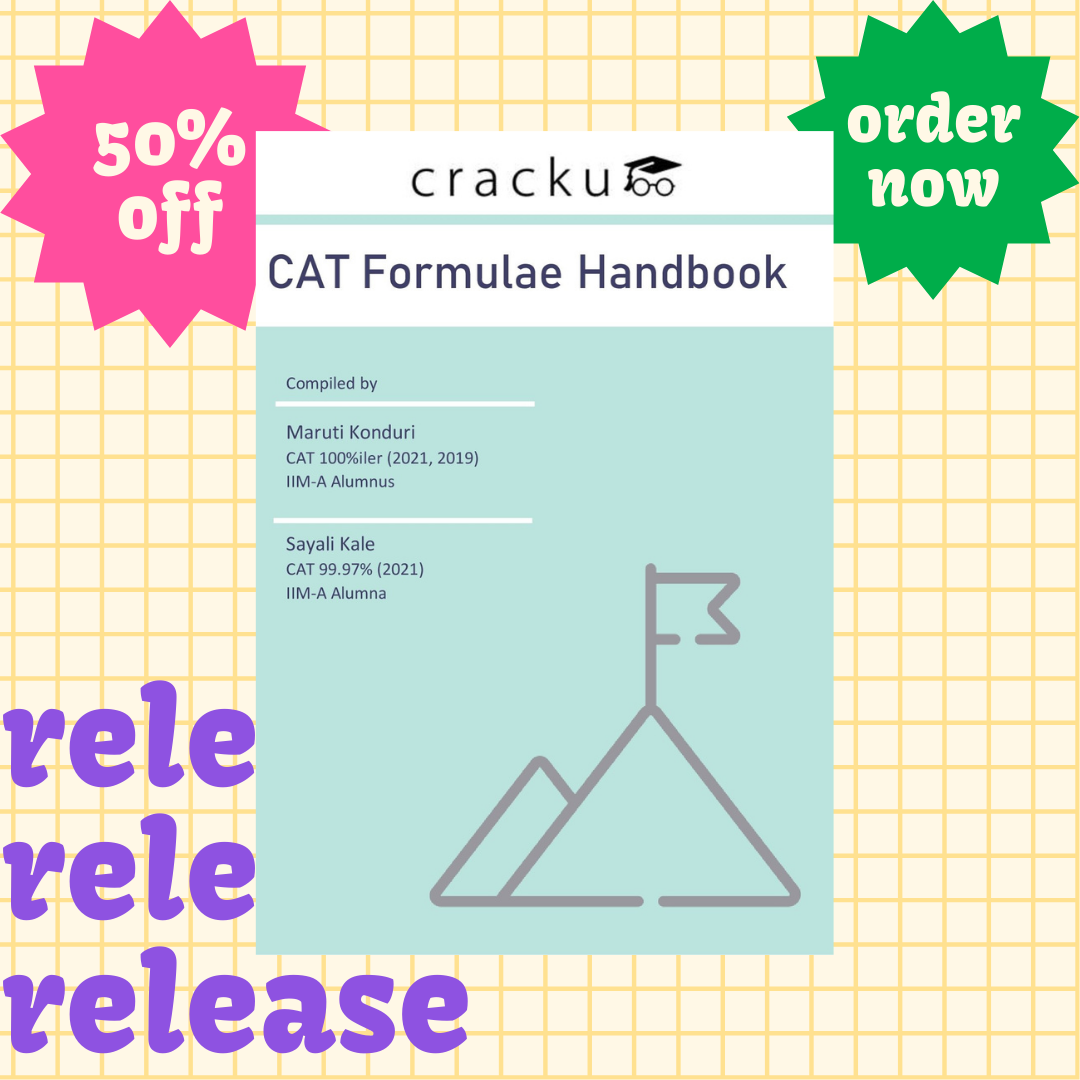# Progressions Formulae For CAT (PDF)

0
52383

Progressions is one of the most scoring topics in CAT and many of the problems can be solved by simple application of formulae. Understanding the concepts is key to avoid making silly mistakes in this. This document contains all the important formulae in progressions for CAT (pdf included).

Looking for hardcopy handbook?

Order below. Delivery charges are on us 🙂The list of concepts covered are:

• Definitions and properties of Arithmetic Progression, Geometric Progression, Harmonic Progression
• Nth term and sum of AP and GP
• Arithmetic Geometric Progression
• Sum of standard series
• Arithmetic Mean, Geometric Mean and Harmonic Mean and their properties

Take 3 free CAT mocks with solutions

Refer to our other important formulae pdfs given below

Number Systems Formulae for CAT (PDF)

Geometry Formulae for CAT (PDF)

Time, Speed and Distance Formulae for CAT (PDF)